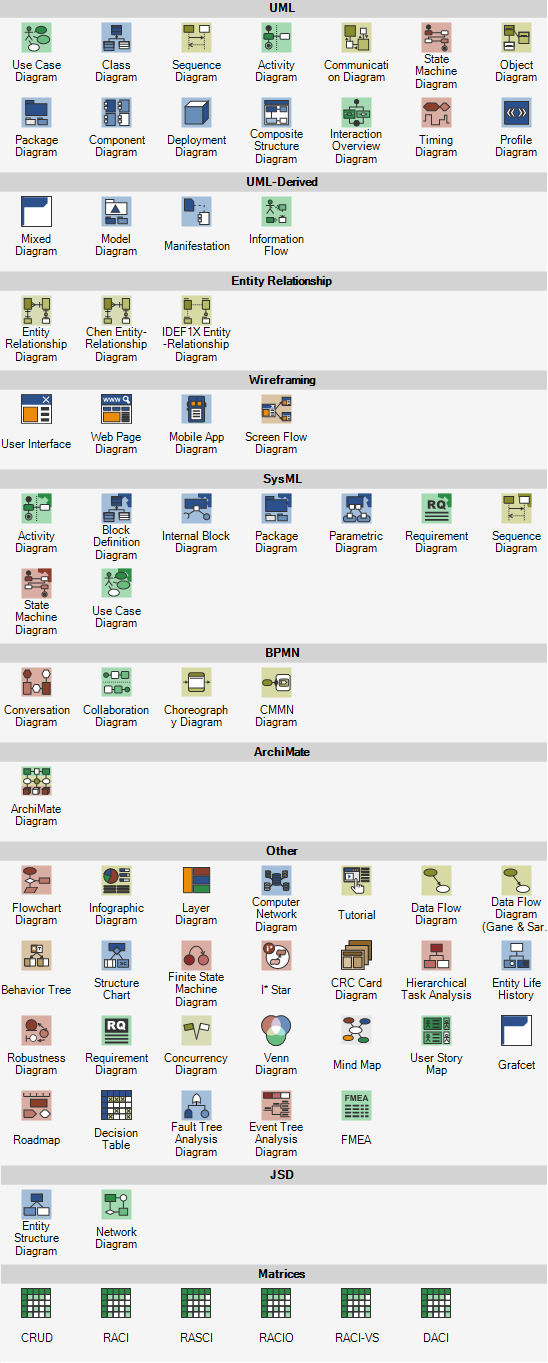# Types of Diagrams and Charts

There are plenty of diagram types you can draw using Software Ideas Modeler. You can depict structures, behavior, processes, workflows, ideas, concepts, and data models with them.

Software Ideas Modeler supports many types of diagrams and charts. This list provides an overview of all supported diagrams, you can create using the diagramming tool.

## SysML Diagram Types

• Activity diagram
• Sequence diagram
• State machine diagram
• Use case diagram
• Block definition diagram
• Internal block diagram
• Parametric diagram
• Package diagram

## JSD Diagrams

• Entity structure diagram
• Network diagram

## Matrices

• CRUD matrix
• RACI matrix
• RASCI matrix
• RACIO matrix
• RACI-VS matrix
• DACI matrix

## Tabular Diagram Types

• Requirement diagram
• Decision table
• Failure mode and effects analysis sheet (FMEA)

## Circular Chart Types

• Pie chart
• Arrowed cycle chart
• Arc chart
• Semicircle chart
• Arc bar chart

## Area Chart Types

• Area bar chart
• Area chart
• Funnel chart
• Line chart
• Point chart
• Line point chart
• Area line chart
• Area line point chart
• Spike chart
• 3D bar chart

## Other Chart Types

• Bar chart
• Table chart
• Spider chart
• Tree map chart

## Other DiagramsTypes of Diagrams

Comment